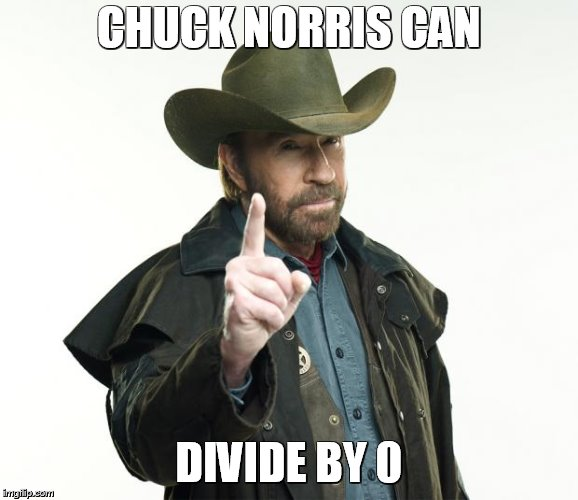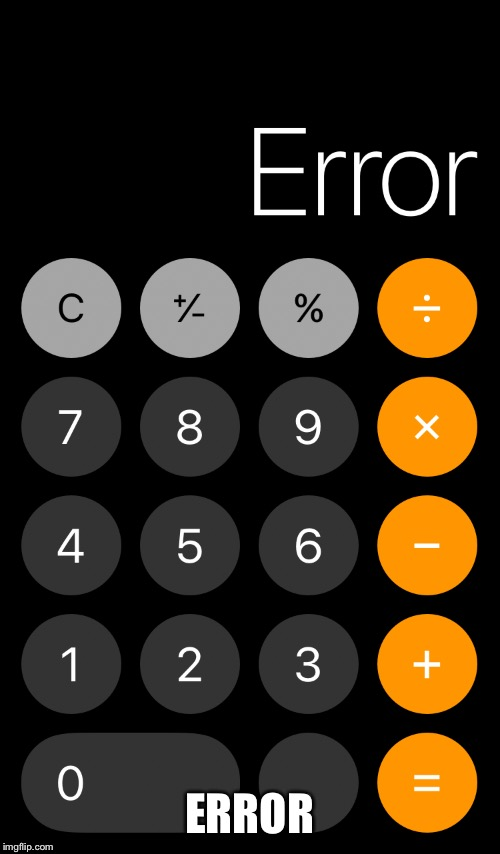I Never Learned This In Algebra!share
 418 views, 5 upvotes, Made by aragorn13 1 year ago memesphilosoraptormathisweirdCaption this Meme
Post Comment
Best first
4 ups, 1y,
2 replies
Undefined.

For example, 2 squared is 4.
2 times 2.
2 cubed, 8, 2 times 2 times 2.
2 to the 4th, 16, 2 times 2 times 2 times 2.
2 to the first, 2 divided by 2.
Like this:
2^4 = 2*2*2*2 = 16
2^3 = 2*2*2 = 8
2^2 = 2*2 = 4
2^1 = 2 = 2
It starts going backwards with division.
2^0 = 2÷2 = 1
2^-1 = 2÷2÷2 = 1/2
2^-2 = 2÷2÷2÷2 = 1/4
2^-3 = 2÷2÷2÷2÷2 = 1/8
...

So,
4^0 = 4÷4 = 1
3^0 = 3÷3 = 1
2^0 = 2÷2 = 1
1^0 = 1÷1 = 1
0^0 = 0÷0 = undefined,
because you can't divide by 0
3 ups, 1y<<<This guy can
1 up, 1yOh.Caption this Meme